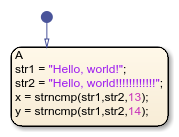# strncmp

Compare first `n` characters of strings (case sensitive)

## Syntax

``tf = strncmp(s1,s2,n)``

## Description

````tf = strncmp(s1,s2,n)` compares up to `n` characters of `s1` and `s2`. The function returns `1` (`true`) if the two are identical and `0` (`false`) otherwise. Text is considered identical if the content of each is the same up to the end or the first `n` characters, whichever comes first.```

Note

The operator `strncmp` is supported only in Stateflow® charts that use MATLAB® as the action language.

## Examples

expand all

Return `1(true)` if the two strings are identical.

```h = "Hello, world!" h2 = "Hello, world!!!!!!!!!!!!" x = strncmp(h,h2,13) x2 = strncmp(h,h2,14)```## Version History

Introduced in R2021b